# OBB碰撞及四叉树优化

1 篇文章 0 订阅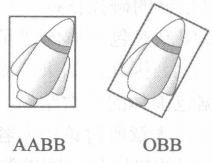## AABB碰撞检测

1.AABB构造：

①中心点加宽高
②者对角线两个点。（这是小学还是初中数学知识记不清了，确定一个矩形的方法）。

球形包围盒：圆心center，半径R


2.AABB相交；
①矩形与矩形相交：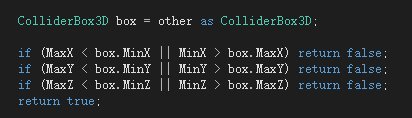②矩形与球形相交：


 if (other.Shape == ShapeType.Sphere)
{
ColliderSphere sphere = other as ColliderSphere;

FixPoint x = sphere.Position.x;
FixPoint y = sphere.Position.y;
FixPoint z = sphere.Position.z;

if (x > MaxX) { x = MaxX; }
if (x < MinX) { x = MinX; }

if (y > MaxY) { y = MaxY; }
if (y < MinY) { y = MinY; }

if (z > MaxZ) { z = MaxZ; }
if (z < MinZ) { z = MinZ; }

FixPoint distance = (x - sphere.Position.x) * (x - sphere.Position.x) +
(y - sphere.Position.y) * (y - sphere.Position.y) +
(z - sphere.Position.z) * (z - sphere.Position.z);

{
return true;
}
return false;
}


③圆与圆相交：无需多言

if(other.Shape == ShapeType.Sphere)
{
Vector3FP other_pos = other.Position;
}


AABB相交到此结束

## OBB构造：

①8个顶点
②6个面
③3组平行面
④一个顶点和三个彼此正交的变相量
⑤中心点，旋转矩阵，3个1/2边长

–《实时检测碰撞算法技术》第4.4章

## OBB相交：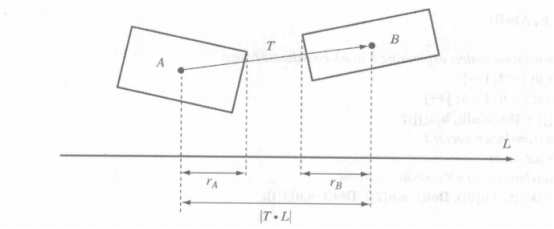下面列出c#代码（刚开始做的时候没有想太多，在网上扒了一份，自己得代码就不贡献了，我没有共享精神，写这个博客也记录一下这个工作）.

public class ObbNew : MonoBehaviour
{
[HideInInspector]
public Transform m_Transform;
[HideInInspector]
public BoxCollider m_BoxCollider;
[HideInInspector]
private float m_Rotation;
[HideInInspector]
public Vector2 m_Extents;
[HideInInspector]
public Vector2[] m_Axiss;

void Start()
{
m_Transform = this.transform;
m_BoxCollider = m_Transform.GetComponent<BoxCollider>();
m_Axiss = new Vector2;
SetExtents();
}

// Update is called once per frame
void Update()
{
m_Rotation = m_Transform.eulerAngles.y * Mathf.PI / 180;

//两个轴
m_Axiss = new Vector2((float)Mathf.Cos(m_Rotation), -(float)Mathf.Sin(m_Rotation));
m_Axiss = new Vector2(Mathf.Sin(m_Rotation), Mathf.Cos(m_Rotation));

}

private void SetExtents()
{
Quaternion rotation = m_Transform.rotation;
m_Transform.rotation = new Quaternion(0, 0, 0, 1);

Vector3 center = m_BoxCollider.center;
Vector3 size = m_BoxCollider.size / 2;

Vector3 Point1 = new Vector3(center.x + size.x, center.y, center.z - size.z);
Vector3 Point2 = new Vector3(center.x - size.x, center.y, center.z + size.z);

Point1 = m_Transform.TransformPoint(Point1);
Point2 = m_Transform.TransformPoint(Point2);

m_Extents = new Vector2(Mathf.Abs(Point1.x - Point2.x) / 2, Mathf.Abs(Point2.z - Point1.z) / 2);
m_Transform.rotation = rotation;

}

public float dot(Vector2 a, Vector2 b)
{
return Mathf.Abs(a.x * b.x + a.y * b.y);
}
{
return (m_Extents.x * dot(m_Axiss, axis) + m_Extents.y * dot(m_Axiss, axis));
}

public bool intersects(ObbNew other)
{
Update();
other.Update();
Vector2 distanceVector = new Vector2(m_Transform.position.x - other.m_Transform.position.x, m_Transform.position.z - other.m_Transform.position.z);

Vector2[] checkObbVector2s =
{
m_Axiss,
m_Axiss,
other.m_Axiss,
other.m_Axiss,

};

for (int index = 0; index < checkObbVector2s.Length; index++)
{
Vector2 curVector2 = checkObbVector2s[index];
{
return false;
}
}
return true;
}

}


//给定点p，返回点q在（或在）OBB b上，最接近p
Vector2 ClosedPtPointToOBB(Point p,OBB b)
{
Vector q
Vector2 d = p -b.center

q = b.center
//2d只考虑x,y  3d则改为3个轴
//遍历 OBB的 轴
for(i = 0; i< 2;i++)
{
//把d投影到轴上，得到距离
//从盒子中心沿d轴
float dis = Vector2.Dot(d,b.u[i])
If(dis >b.e[i] )
Dis = b.e[i]
If(dis < -b.e[i])
dis = -b.e[i]

q +=dis * b.u[i]

}
return q
}

Test SphereOBB(Sphere s, OBB b )
{
Vector2 p = ClosedPtPointToOBB(s.center,b)
Vector2 v = p - s.center

}


1.在obj插入到四叉树中时，会有象限判断，计算符合该obj插入象限的位置，总共四个象限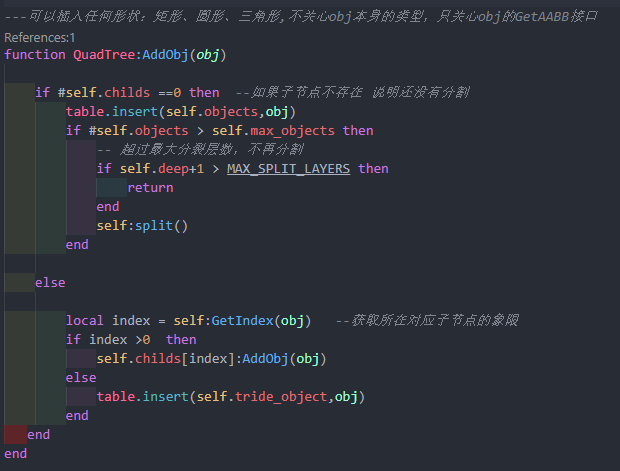可以插入任何形状：矩形、圆形、三角形,四叉树不关心obj本身的类型，只关心obj的GetAABB接口

1.三角形是否属于这个象限，三角形三个顶点都在这个象限，则该三角形属于这个象限
2.矩形是够属于这个象限，矩形的最大顶点和最小顶点是否在同一象限（对角线顶点）
3.圆是够在这个象限，圆心与矩形中心的距离与半径判断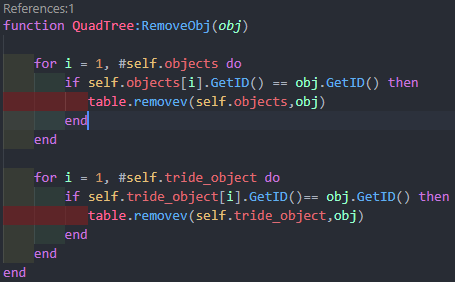在编写博客的时候顺便优化了下，原来是if self.objects[i].collider.entityid == obj.collider.entityid then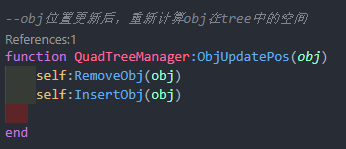1.游戏初始化构造四叉树，深度定为8-10（综合项目地图和物体，这个深度需要根据不同情况变化）
2.实例化物体碰撞时，同步添加一份碰撞信息到树中。
3随着物体增多，树不断分裂
4.物体移动时，更新所在区域
5.检测碰撞时，找到这个物体可能碰撞的其余物体，四叉树递归
6.根据物体标签再进一步筛选可碰撞的list
7.碰撞检测.

## 总结：

1.写代码之前不设计，整个实现过程很慢，很浪费时间，如果在前***期设计规划一下，会很快的实现***。
2.数学呀！数学！
3.代码写完要优化，或者写的时候就要考虑到优化。我一直不信邪，一直被告诫c#和lua相互传递会很浪费效率，我就不以为意。直到500个碰撞体一起检测那一刹那，我的帧率快爆了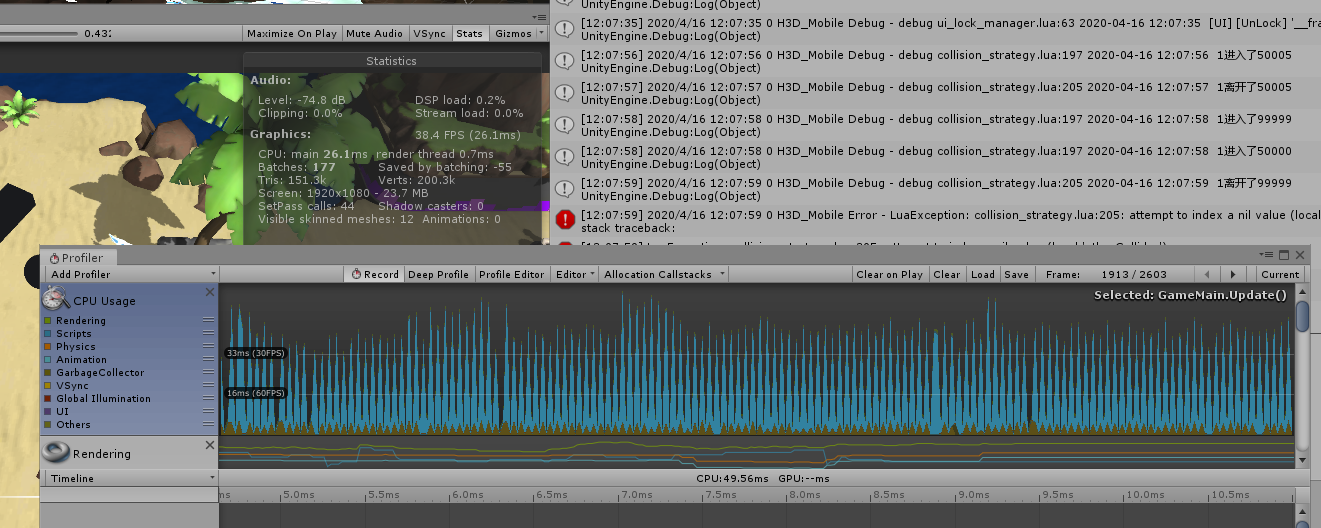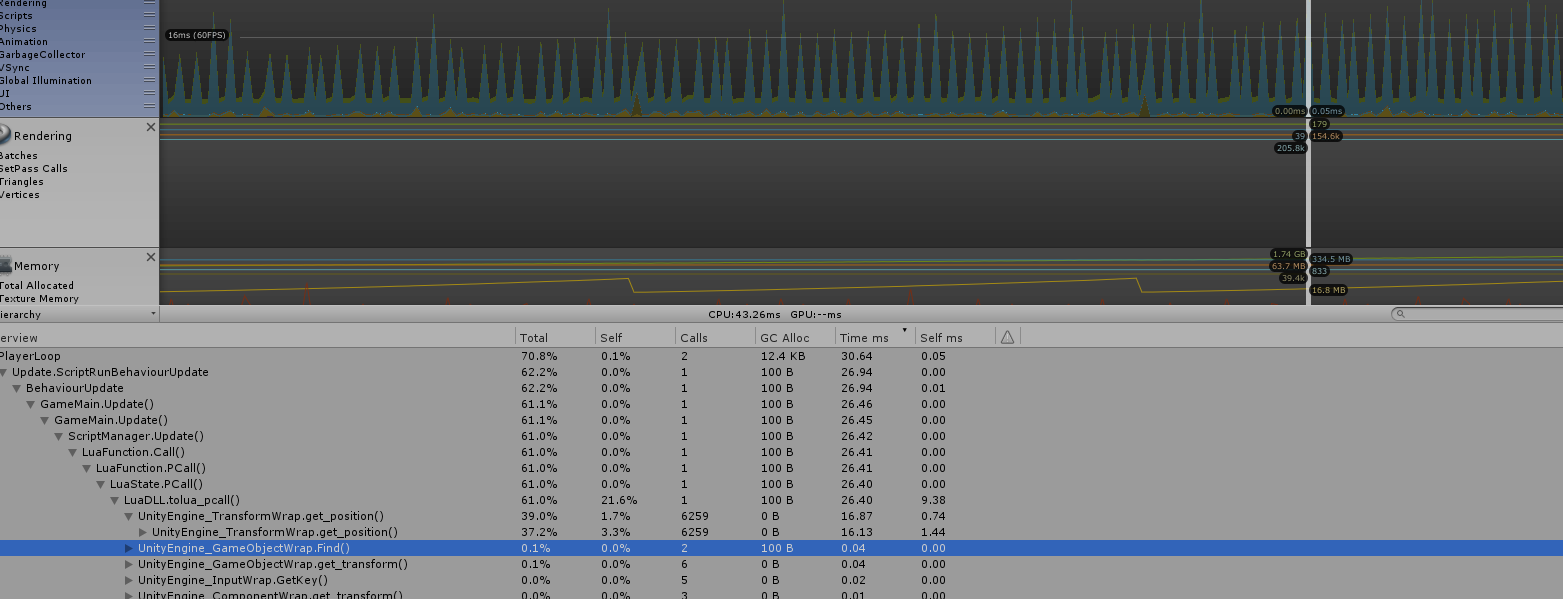Entity通过Proto数据创建碰撞体，不要访问GameObject，碰撞体有自身相对的长宽高数据，再根据LogicTransform的Position，Rotation算出对应的顶点

10-1012-25
12-313773
01-052703
03-197606
02-08215
01-24395
03-251万+
05-152863
01-251万+
01-311260
08-031万+
06-263070点击重新获取扫码支付余额充值